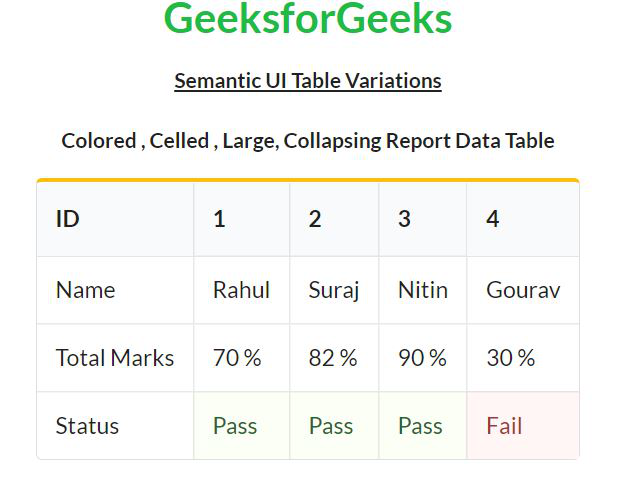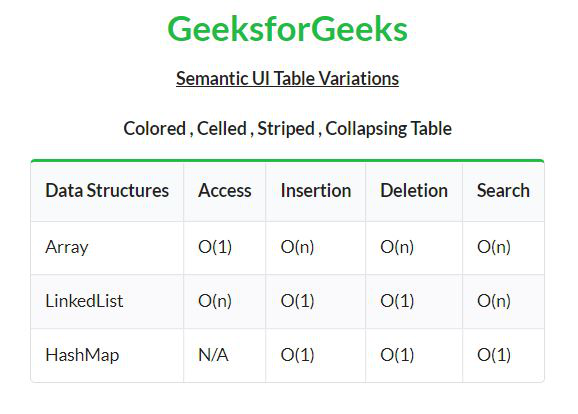Open in App
Not now

# Semantic-UI Table Variations

• Last Updated : 16 May, 2022

Semantic UI is an open-source framework that uses CSS and jQuery to build great user interfaces. It is the same as a bootstrap for use and has great different elements to use to make your website look more amazing.

Semantic UI has a bunch of components for user interface design. One of them is the Table component. Tables are an easy way to organize a lot of data. A table is an arrangement of data in rows and columns, or possibly in a more complex structure. There are different variations of tables available in Semantic UI.

Semantic UI Table Variations:

• Single line: This variation is used to display table content on a single line.
• Fixed: This variation does not resize the table according to its content.
• Stacking: This variation is used to stack table content responsively.
• Selectable Row: This variation is used to make a table row or rows appear selected.
• Selectable Cell:  This variation is used to make the table cell appear selected.
• Vertical Alignment: This variation allows the user to align table content vertically as per his requirement.
• Text Alignment: This variation allows the user to align table content horizontally as per his requirement.
• Striped: This variation creates a table with contrasting colors to alternate rows.
• Celled: This variation is used to compose each row into different cells.
• Basic: This variation is used to create simple tables with very less styling to increase readability.
• Collapsing Cell: This variation is used to collapse table cell size equal to its content.
• Column Count:  This variation allows the user to define the table column count beforehand.
• Column Width: This variation is used to set table’s individual column’s width.
• Collapsing: This variation is used to create tables with space equal to the number of rows defined.
• Colored: This variation is used to give different colors to tables.
• Inverted: This variation is used to invert the table color.
• Sortable: This variation allows the user to sort a table’s content by clicking on its header.
• Full-Width Header / Footer: This variation is used to define the full-width header/footer to the table.
• Padded: This variation is used to increase row padding to make rows more visible.
• Compact: This variation is used to reduce row padding to make more rows visible on a single page.
• Size: This variation is used to create small or large tables.

Syntax:

```<table class="Table Variations Class">
<tr>
<td>...</td>
</tr>
...
</table>```

The below example illustrates the different variations of tables.

Example 1: This example describes some of the table variations in Semantic UI.

## HTML

 ` ` `<``html``> ` ` `  `<``head``> ` `    ``<``title``>Semantic UI Table Variation ` `    ``<``link` `href``= ` `"https://cdnjs.cloudflare.com/ajax/libs/semantic-ui/2.4.1/semantic.min.css"`  `          ``rel``=``"stylesheet"` `/>     ` ` ` ` `  `<``body``> ` `    ``<``center``> ` `        ``<``h1` `class``=``"ui green header"``>GeeksforGeeks ` `        ``<``strong``> ` `              ``<``u``>Semantic UI Table Variations ` `          `` ` `          ``<``br` `/><``br` `/> ` `        ``<``strong``> ` `            ``Colored, Celled, Large, Collapsing  ` `            ``Report Data Table ` `        `` ` `        ``<``table` `class``=``"ui yellow celled large collapsing table"``> ` `            ``<``thead``> ` `                ``<``tr``> ` `                    ``<``th``>ID ` `                    ``<``th``>1 ` `                    ``<``th``>2 ` `                    ``<``th``>3 ` `                    ``<``th``>4 ` `                `` ` `            `` ` `            ``<``tbody``> ` `                ``<``tr``> ` `                    ``<``td``>Name ` `                    ``<``td``>Rahul ` `                    ``<``td``>Suraj ` `                    ``<``td``>Nitin ` `                    ``<``td``>Gourav ` `                `` ` `                ``<``tr``> ` `                    ``<``td``>Total Marks ` `                    ``<``td``>70 % ` `                    ``<``td``>82 % ` `                    ``<``td``>90 % ` `                    ``<``td``>30 % ` `                `` ` `                ``<``tr``> ` `                    ``<``td` `>Status ` `                    ``<``td` `class``=``"positive"``>Pass ` `                    ``<``td` `class``=``"positive"``>Pass ` `                    ``<``td` `class``=``"positive"``>Pass ` `                    ``<``td` `class``=``"negative"``>Fail ` `                `` ` `            `` ` `        `` ` `    `` ` ` ` ``

Output:Table Variations

Example 2: This example describes some of the table variations in Semantic UI.

## HTML

 ` ` `<``html``> ` ` `  `<``head``> ` `    ``<``title``>Semantic UI Table Variation ` `    ``<``link` `href``= ` `"https://cdnjs.cloudflare.com/ajax/libs/semantic-ui/2.4.1/semantic.min.css"`  `          ``rel``=``"stylesheet"` `/>     ` ` ` ` `  `<``body``> ` `    ``<``center``> ` `        ``<``h1` `class``=``"ui green header"``>GeeksforGeeks ` `        ``<``strong``> ` `              ``<``u``>Semantic UI Table Variations ` `          `` ` `          ``<``br` `/><``br` `/> ` `        ``<``strong``> ` `            ``Colored, Celled, Striped, Collapsing Table ` `        `` ` `        ``<``table` `class``=``"ui green celled collapsing striped table"``> ` `            ``<``thead``> ` `                ``<``tr``> ` `                    ``<``th``>Data Structures ` `                    ``<``th``>Access ` `                    ``<``th``>Insertion ` `                    ``<``th``>Deletion ` `                    ``<``th``>Search ` `                `` ` `            `` ` `            ``<``tbody``> ` `                ``<``tr``> ` `                    ``<``td``>Array ` `                    ``<``td``>O(1) ` `                    ``<``td``>O(n) ` `                    ``<``td``>O(n) ` `                    ``<``td``>O(n) ` `                `` ` `                ``<``tr``> ` `                    ``<``td``>LinkedList ` `                    ``<``td``>O(n) ` `                    ``<``td``>O(1) ` `                    ``<``td``>O(1) ` `                    ``<``td``>O(n) ` `                `` ` `                ``<``tr``> ` `                    ``<``td``>HashMap ` `                    ``<``td``>N/A ` `                    ``<``td``>O(1) ` `                    ``<``td``>O(1) ` `                    ``<``td``>O(1) ` `                `` ` `            `` ` `        `` ` `    `` ` ` ` ``

Output:Table Variations

My Personal Notes arrow_drop_up
Related Articles